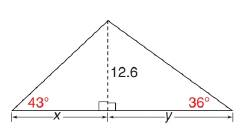Chapter 11.3, Problem 46EElementary Geometry For College St...

7th Edition
Alexander + 2 others
ISBN: 9781337614085

Solutions

Chapter
SectionElementary Geometry For College St...

7th Edition
Alexander + 2 others
ISBN: 9781337614085
Textbook Problem

In the triangle shown, find each measure to the nearest tenth of a unit.a) x b) y c) A , the area of the triangleTo determine

a)

The value of side x to the nearest tenth of a unit.

Explanation

Given:

The given figure is shown below:

Calculation:

The triangles ABC are right triangles.

According to the definition of tangent ratio, tan(mBCA)=ABBC

tan(mBCA)=ABBC=12

To determine

b)

The value of side y to the nearest tenth of a unit.

To determine

c)

The area of the triangle of the given figure.

Still sussing out bartleby?

Check out a sample textbook solution.

See a sample solution

The Solution to Your Study Problems

Bartleby provides explanations to thousands of textbook problems written by our experts, many with advanced degrees!

Get Started

(x3y2z2)2

Applied Calculus for the Managerial, Life, and Social Sciences: A Brief Approach

In problems 1-16, solve each equation. 5. Solve

Mathematical Applications for the Management, Life, and Social Sciences

What is the defining characteristic of a repeated-measures or within-subjects research design?

Essentials of Statistics for The Behavioral Sciences (MindTap Course List)

True or False: is conservative.

Study Guide for Stewart's Multivariable Calculus, 8th

Evaluate .

Study Guide for Stewart's Single Variable Calculus: Early Transcendentals, 8th